# Using Fastai and TensorFlow.js for Hand Gesture Recognition in Unity Pt. 1

fastai
unity
tensorflow
webgl
tutorial
Train a hand gesture classifier using fastai and export it to TensorFlow.js.
Author

Christian Mills

Published

October 4, 2022

## Introduction

In this three-part tutorial series, we will use fastai and TensorFlow.js to create an in-browser hand gesture recognition system in Unity. In Part 1, we will train a hand gesture classifier using fastai and export it to TensorFlow.js. In Part 2, we will create a TensorFlow.js plugin for the Unity game engine. Finally, in Part 3, we will host the Unity project as a live demo on GitHub Pages. By the end of this tutorial series, you will have a hand gesture recognition system that you can use in your Unity projects.

In-Browser Demo: Hand Gesture Classifier

The tutorial uses a downscaled version of HaGRID (HAnd Gesture Recognition Image Dataset) that I modified for image classification tasks. The dataset contains images for 18 distinct hand gestures and an additional `no_gesture` class to account for idle hands.

Reference Images
Class Image
calldislikefistfour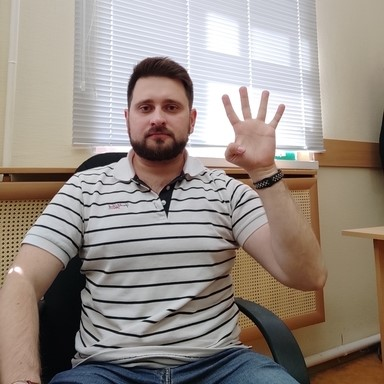likemuteokonepalm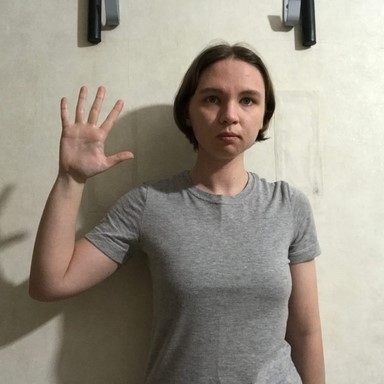peace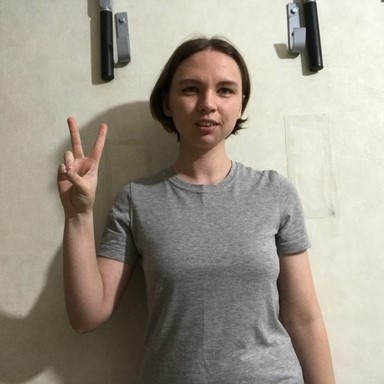peace_invertedrockstop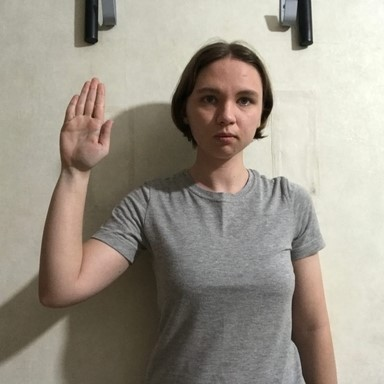stop_invertedthree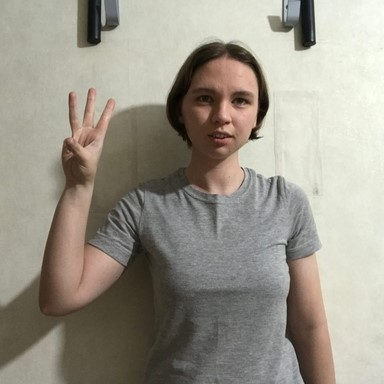three2two_uptwo_up_invertedWe can use a model trained on this dataset to map hand gestures to user input via a webcam in Unity.

## Overview

In Part 1 of this tutorial series, we finetune an image classifier from the timm library using fastai and export it to TensorFlow.js. We will start by installing and importing the necessary dependencies. Then, we will select a model to use and download a dataset to train it. After inspecting the dataset, we will define data loaders to use for training. Finally, we will finetune and export the model. We also demonstrate how to clean the dataset to improve training. By the end of this post, you will have a trained hand gesture classifier that you can use in web applications.

You can find links to view the training code and run it on Google Colab and Kaggle below.

Jupyter Notebook Colab Kaggle
GitHub Repository Open In Colab Open in Kaggle

## Install Dependencies

The training code requires PyTorch for the fastai library, the fastai library itself for training, and the Kaggle API Python package for downloading the dataset. The timm library provides access to a wide range of pretrained image models.

Install model training dependencies

``````# %%capture
# !pip install -U torch torchvision torchaudio
# !pip install -U fastai==2.7.9
# !pip install -U kaggle
# !pip install -U Pillow
# !pip install -U timm``````

The model conversion process involves exporting the PyTorch model to ONNX, converting the ONNX model to a TensorFlow SavedModel, then converting the SavedModel to TensorFlow.js web format.

Install Tensorflow.js conversion dependencies

``````# %%capture
# !pip install -U onnxruntime
# !pip install onnx-tf
# !pip install tensorflowjs
# !pip install tensorflow_probability
# !pip install onnx-simplifier
# !pip install simple-onnx-processing-tools
# !pip install -U onnx_graphsurgeon --index-url https://pypi.ngc.nvidia.com``````

## Import Dependencies

Import all fastai computer vision functionality

``from fastai.vision.all import *``

Import pandas and disable column and row limits

``````import pandas as pd
pd.set_option('max_colwidth', None)
pd.set_option('display.max_rows', None)
pd.set_option('display.max_columns', None)``````

Import timm library

``import timm``
``timm.__version__``
``'0.6.7'``

## Select a Model

Timm provides many pretrained models, but not all are fast enough for real-time applications. We can filter through the available models using the `timm.list_models()` function.

View available ResNet models

``pd.DataFrame(timm.list_models('resnet*', pretrained=True))``
0
0 resnet10t
1 resnet14t
2 resnet18
3 resnet18d
4 resnet26
5 resnet26d
6 resnet26t
7 resnet32ts
8 resnet33ts
9 resnet34
10 resnet34d
11 resnet50
12 resnet50_gn
13 resnet50d
14 resnet51q
15 resnet61q
16 resnet101
17 resnet101d
18 resnet152
19 resnet152d
20 resnet200d
21 resnetaa50
22 resnetblur50
23 resnetrs50
24 resnetrs101
25 resnetrs152
26 resnetrs200
27 resnetrs270
28 resnetrs350
29 resnetrs420
30 resnetv2_50
31 resnetv2_50d_evos
32 resnetv2_50d_gn
33 resnetv2_50x1_bit_distilled
34 resnetv2_50x1_bitm
35 resnetv2_50x1_bitm_in21k
36 resnetv2_50x3_bitm
37 resnetv2_50x3_bitm_in21k
38 resnetv2_101
39 resnetv2_101x1_bitm
40 resnetv2_101x1_bitm_in21k
41 resnetv2_101x3_bitm
42 resnetv2_101x3_bitm_in21k
43 resnetv2_152x2_bit_teacher
44 resnetv2_152x2_bit_teacher_384
45 resnetv2_152x2_bitm
46 resnetv2_152x2_bitm_in21k
47 resnetv2_152x4_bitm
48 resnetv2_152x4_bitm_in21k

The smaller ResNet models are both fast and sufficiently accurate in most settings. Unfortunately, the `resnet10t` and `resnet14t` models contain operations unsupported by the TensorFlow.js conversion script. We’ll instead use the `resnet18` model for our lightweight option.

Inspect config for specific model

Each model comes with a set of default configuration parameters. We must keep track of the `mean` and `std` values used to normalize the model input. Many pretrained models use the ImageNet normalization stats, but others, like MobileViT, do not.

``````from timm.models import resnet
resnet_model = 'resnet18'
pd.DataFrame.from_dict(resnet.default_cfgs[resnet_model], orient='index')``````
0
num_classes 1000
input_size (3, 224, 224)
pool_size (7, 7)
crop_pct 0.875
interpolation bilinear
mean (0.485, 0.456, 0.406)
std (0.229, 0.224, 0.225)
first_conv conv1
classifier fc

View available ConvNeXt models

``pd.DataFrame(timm.list_models('convnext*', pretrained=True))``
0
0 convnext_base
1 convnext_base_384_in22ft1k
2 convnext_base_in22ft1k
3 convnext_base_in22k
4 convnext_large
5 convnext_large_384_in22ft1k
6 convnext_large_in22ft1k
7 convnext_large_in22k
8 convnext_nano
9 convnext_small
10 convnext_small_384_in22ft1k
11 convnext_small_in22ft1k
12 convnext_small_in22k
13 convnext_tiny
14 convnext_tiny_384_in22ft1k
15 convnext_tiny_hnf
16 convnext_tiny_in22ft1k
17 convnext_tiny_in22k
18 convnext_xlarge_384_in22ft1k
19 convnext_xlarge_in22ft1k
20 convnext_xlarge_in22k

The `convnext_nano` model is highly accurate for its size and is a good choice when compute power is less constrained.

Inspect config for specific model

``````from timm.models import convnext
convnext_model = 'convnext_nano'
pd.DataFrame.from_dict(convnext.default_cfgs[convnext_model], orient='index')``````
0
num_classes 1000
input_size (3, 224, 224)
pool_size (7, 7)
crop_pct 0.95
interpolation bicubic
mean (0.485, 0.456, 0.406)
std (0.229, 0.224, 0.225)
first_conv stem.0
test_input_size (3, 288, 288)
test_crop_pct 1.0

Select a model

``````# model_type = resnet
# model_name = resnet_model``````
``````model_type = convnext
model_name = convnext_model``````

Extract normalization stats from model config

``````mean = model_type.default_cfgs[model_name]['mean']
std = model_type.default_cfgs[model_name]['std']
mean, std``````
``((0.485, 0.456, 0.406), (0.229, 0.224, 0.225))``

The Kaggle API tool requires an API Key for a Kaggle account. Sign in or create a Kaggle account using the link below, then click the Create New API Token button.

Kaggle will generate and download a `kaggle.json` file containing your username and new API token. Initialize the `creds` variable with the values for each.

Enter Kaggle username and API token

``creds = '{"username":"","key":""}'``

Save Kaggle credentials if none are present

``````cred_path = Path('~/.kaggle/kaggle.json').expanduser()
# Save API key to a json file if it does not already exist
if not cred_path.exists():
cred_path.parent.mkdir(exist_ok=True)
cred_path.write_text(creds)
cred_path.chmod(0o600)``````

Import Kaggle API

``from kaggle import api``

Select a dataset

Now that we have our Kaggle credentials set, we need to define the dataset and where to store it. I made three different-sized versions of the dataset available on Kaggle.

``````dataset_name = 'hagrid-classification-512p-no-gesture-150k'
# dataset_name = 'hagrid-classification-512p-no-gesture-300k'
# dataset_name = 'hagrid-classification-512p-no-gesture'

kaggle_dataset = f'innominate817/{dataset_name}'``````

Define path to dataset

We will use the default archive and data folders for the fastai library to store the compressed and uncompressed datasets.

``````archive_dir = URLs.path()
dataset_dir = archive_dir/'../data'
archive_path = Path(f'{archive_dir}/{dataset_name}.zip')
dataset_path = Path(f'{dataset_dir}/{dataset_name}')``````

Define method to extract the dataset from an archive file

``````def file_extract(fname, dest=None):
"Extract `fname` to `dest` using `tarfile` or `zipfile`."
if dest is None: dest = Path(fname).parent
fname = str(fname)
if   fname.endswith('gz'):  tarfile.open(fname, 'r:gz').extractall(dest)
elif fname.endswith('zip'): zipfile.ZipFile(fname     ).extractall(dest)
else: raise Exception(f'Unrecognized archive: {fname}')``````

``````if not archive_path.exists():
file_extract(fname=archive_path, dest=dataset_path)``````

## Inspect the Dataset

Inspect the dataset path

``pd.DataFrame(list(dataset_path.ls()))``
0
0 /home/innom-dt/.fastai/archive/../data/hagrid-classification-512p-no-gesture-150k/hagrid-classification-512p-no-gesture-150k

Get image file paths

``img_dir = dataset_path/dataset_name``
``````files = get_image_files(img_dir)
len(files)``````
``154816``

Inspect files

The dataset indicates the image class in the parent folder names.

``pd.DataFrame([files, files[-1]])``
0
0 /home/innom-dt/.fastai/archive/../data/hagrid-classification-512p-no-gesture-150k/hagrid-classification-512p-no-gesture-150k/stop/3ac51cf4-cd81-4803-a608-76a55b36df26.jpeg
1 /home/innom-dt/.fastai/archive/../data/hagrid-classification-512p-no-gesture-150k/hagrid-classification-512p-no-gesture-150k/two_up/d5a0a30d-92aa-4a7c-9621-1fed0e8f0b66.jpeg

Inspect class folder names

There are 19 class folders, and the dataset does not predefine a training-validation split.

``````folder_names = [path.name for path in Path(img_dir).ls()]
if 'models' in folder_names:
os.removedirs(img_dir/'models')
folder_names.remove('models')
folder_names.sort()
print(f"Num classes: {len(folder_names)}")
pd.DataFrame(folder_names)``````
``Num classes: 19``
0
0 call
1 dislike
2 fist
3 four
4 like
5 mute
6 no_gesture
7 ok
8 one
9 palm
10 peace
11 peace_inverted
12 rock
13 stop
14 stop_inverted
15 three
16 three2
17 two_up
18 two_up_inverted

Inspect one of the training images

``````import PIL
img = PIL.Image.open(files)
print(f"Class: {files.parent.name}")
print(f"Image Dims: {img.shape}")
img``````
``````    Class: stop
Image Dims: (512, 512)``````

Next, we need to define the Transforms for the DataLoaders object.

Define target input dimensions

The Unity project will take input from a webcam, which will likely not have a square aspect ratio. We can still train the models with a square aspect ratio, and training at `256x256` (65,536 pixels) is more efficient than training at `384x216` (82,944 pixels) for a 16:9 aspect ratio.

The ResNet and ConvNeXt models handle arbitrary input dimensions well. However, we must export some models like MobileViT with the exact input dimensions used for inference.

``````size_1_1 = (256, 256)
size_16_9 = (216, 384)
size = size_1_1``````

Define Transforms

We can leave most of the options in `aug_transforms` at their default values. The HaGRID dataset is diverse enough that we don’t need to add too much augmentation. However, we will disable the `max_rotate` option as orientation is relevant for gesture recognition.

``````item_tfms = [Resize(size, method=ResizeMethod.Pad, pad_mode=PadMode.Border)]

batch_tfms = [
*aug_transforms(
size=size,
mult=1.0,
do_flip=True,
flip_vert=False,
max_rotate=0.0,
min_zoom=1.0,
max_zoom=1.1,
max_lighting=0.2,
max_warp=0.2,
p_affine=0.75,
]``````

Note: The fastai library automatically normalizes input for timm models as of version 2.7.5.

Define batch size

``bs = 32``

We can use the `from_folder` method to instantiate the DataLoaders object.

``````dls = ImageDataLoaders.from_folder(
path=img_dir,
valid_pct=0.2,
bs=bs,
item_tfms=item_tfms,
batch_tfms=batch_tfms
)``````

Let’s verify the DataLoaders object works as expected before training a model.

``dls.train.show_batch()``

## Finetune the Model

Now we can define the `Learner` object and finetune the selected model.

Define metrics

``metrics = [error_rate, accuracy]``

Define Learner object

``learn = vision_learner(dls, model_name, metrics=metrics)``

Define model checkpoint file path

``````checkpoint_path = Path(f"{dataset_path.name}-{model_name}.pth")
checkpoint_path``````
``Path('hagrid-classification-512p-no-gesture-150k-convnext_nano.pth')``

``````# if checkpoint_path.exists():

Find learning rate

``suggested_lrs = learn.lr_find()``

Define learning rate

We can use a slightly higher learning rate than the learning rate finder recommends to speed up training.

``````lr = suggested_lrs.valley*3
lr``````
``0.003606793354265392``

Define number of epochs

We should not need to train for more than a few epochs.

``epochs = 4``

Define callbacks

Training with mixed precision can significantly reduce training time on modern GPUs. However, the older GPUs on the free tiers for Google Colab and Kaggle will likely not benefit from it.

``cbs = [MixedPrecision()]``

Fine tune model

``learn.fine_tune(epochs, base_lr=lr, cbs=cbs)``
epoch train_loss valid_loss error_rate accuracy time
0 0.372442 0.189683 0.061299 0.938701 04:22
epoch train_loss valid_loss error_rate accuracy time
0 0.096614 0.054749 0.017214 0.982786 05:29
1 0.048555 0.033598 0.010012 0.989988 05:34
2 0.030899 0.018264 0.005555 0.994445 05:32
3 0.018128 0.015447 0.004877 0.995123 05:30

Save model checkpoint

``torch.save(learn.model.state_dict(), checkpoint_path)``

Make predictions for a group of images

``learn.show_results()``

Define an Interpretation object

Once the model finishes training, we can create an Interpretation object to see where it struggles. An Interpretation object is also helpful to see if there are any mislabeled/low-quality training images.

``interp = Interpretation.from_learner(learn)``

Plot top losses

``interp.plot_top_losses(k=9, figsize=(15,10))``

## Clean Dataset (Optional)

I spent some time cleaning the modified HaGRID datasets, but there are likely some training images that should still be moved or deleted. If the model accuracy is insufficient, consider using the `ImageClassifierCleaner` widget to edit the dataset.

Import fastai ImageClassifierCleaner

``# from fastai.vision.widgets import ImageClassifierCleaner``

Mark images to delete or move

``````# cleaner = ImageClassifierCleaner(learn)
# cleaner``````

Inspect samples to change

``# cleaner.change()``

Move selected samples to target class folder

``# for idx,cat in cleaner.change(): shutil.move(str(cleaner.fns[idx]), img_dir/cat)``

Inspect samples to delete

``# cleaner.delete()``

Delete selected samples

``# for idx in cleaner.delete(): cleaner.fns[idx].unlink()``

Note: Restart the notebook and finetune the trained model after cleaning the dataset.

## Test the Model

Next, we will test the model on a single image.

Select a test image

``import PIL``
``````test_file = files
test_file.parent.name, test_file.name``````
``('stop', '3ac51cf4-cd81-4803-a608-76a55b36df26.jpeg')``
``````test_img = PIL.Image.open(test_file)
print(f"Source image size: {test_img.size}")
test_img``````
``Source image size: (512, 512)``

Set target size

We will test the model with the target inference resolution to verify it performs as desired.

``````size = size_16_9
size``````
``(216, 384)``

Option 1: Pad to target input dims

``````padded_img = PIL.ImageOps.pad(test_img, [size, size], method=PIL.Image.Resampling.BICUBIC)
``Padded image size: (384, 216)``

Option 2: Stretch to target input dims

``````stretched_img = test_img.resize([size, size])
print(f"Stretched image size: {stretched_img.size}")
stretched_img``````
``Stretched image size: (384, 216)``

Make a prediction on padded image using a fastai.vision.core.PILImage

``````pred = learn.predict(PILImage(padded_img))
pred, pred.max()``````
``````    (('stop',
TensorBase(13),
TensorBase([5.6481e-08, 2.9167e-09, 1.7013e-08, 1.0619e-07, 7.0342e-09, 4.3362e-10,
1.9056e-08, 7.8481e-07, 1.9450e-08, 5.0064e-06, 3.8272e-09, 8.2519e-11,
4.3597e-08, 9.9999e-01, 2.3984e-08, 1.0935e-08, 2.4180e-09, 2.1497e-08,
5.9654e-10])),
TensorBase(1.0000))``````

Make a prediction on stretched image using a fastai.vision.core.PILImage

``````pred = learn.predict(PILImage(stretched_img))
pred, pred.max()``````
``````(('stop',
TensorBase(13),
TensorBase([1.3940e-06, 4.6373e-07, 1.3970e-04, 6.4621e-07, 6.8510e-08, 9.9468e-09,
1.1748e-07, 1.3881e-06, 1.1672e-06, 3.1076e-04, 9.0491e-09, 7.7261e-10,
8.4245e-08, 9.9954e-01, 1.7898e-07, 2.6569e-08, 3.4712e-08, 2.2750e-08,
6.5716e-09])),
TensorBase(0.9995))``````

When we are satisfied with the model, we can start preparing for implementing it in TensorFlow.js. We will need to apply some of the preprocessing and post-processing that fastai applies automatically.

Inspect the `after_item` pipeline

We do not need to worry about padding the input image as both the ResNet and ConvNeXt models handle arbitrary input dimensions.

``learn.dls.after_item``
``Pipeline: Resize -- {'size': (256, 256), 'method': 'pad', 'pad_mode': 'border', 'resamples': (<Resampling.BILINEAR: 2>, <Resampling.NEAREST: 0>), 'p': 1.0} -> ToTensor``

Inspect the `after_batch` pipeline

The `after_batch` pipeline first scales the image color channel values from `[0,255]` to `[0,1]`. We will need to do the same for the TensorFlow.js plugin. We will also need to normalize the input image with the relevant normalization stats.

``learn.dls.after_batch``
``````Pipeline: IntToFloatTensor -- {'div': 255.0, 'div_mask': 1} -> Flip -- {'size': (256, 256), 'mode': 'bilinear', 'pad_mode': 'border', 'mode_mask': 'nearest', 'align_corners': True, 'p': 0.5} -> Brightness -- {'max_lighting': 0.2, 'p': 1.0, 'draw': None, 'batch': False} -> Normalize -- {'mean': tensor([[[[0.4850]],

[[0.4560]],

[[0.4060]]]], device='cuda:0'), 'std': tensor([[[[0.2290]],

[[0.2240]],

[[0.2250]]]], device='cuda:0'), 'axes': (0, 2, 3)}``````

Convert image to tensor

We’ll first prepare the input image by converting it to a tensor, batching it, and moving it to the GPU.

``````img_tensor = tensor(padded_img).permute(2, 0, 1).float().unsqueeze(dim=0).cuda()
img_tensor.shape, img_tensor``````
``````    (torch.Size([1, 3, 216, 384]),
tensor([[[[0., 0., 0.,  ..., 0., 0., 0.],
[0., 0., 0.,  ..., 0., 0., 0.],
[0., 0., 0.,  ..., 0., 0., 0.],
...,
[0., 0., 0.,  ..., 0., 0., 0.],
[0., 0., 0.,  ..., 0., 0., 0.],
[0., 0., 0.,  ..., 0., 0., 0.]],

[[0., 0., 0.,  ..., 0., 0., 0.],
[0., 0., 0.,  ..., 0., 0., 0.],
[0., 0., 0.,  ..., 0., 0., 0.],
...,
[0., 0., 0.,  ..., 0., 0., 0.],
[0., 0., 0.,  ..., 0., 0., 0.],
[0., 0., 0.,  ..., 0., 0., 0.]],

[[0., 0., 0.,  ..., 0., 0., 0.],
[0., 0., 0.,  ..., 0., 0., 0.],
[0., 0., 0.,  ..., 0., 0., 0.],
...,
[0., 0., 0.,  ..., 0., 0., 0.],
[0., 0., 0.,  ..., 0., 0., 0.],
[0., 0., 0.,  ..., 0., 0., 0.]]]], device='cuda:0'))``````

Scale tensor values

We’ll then scale the values from [0, 255] to [0, 1].

``scaled_tensor = img_tensor / 255``

Next, we’ll prepare the normalization values.

Prepare mean values

``````mean_tensor = tensor(mean).view(1,1,-1).permute(2, 0, 1).unsqueeze(dim=0).cuda()
mean_tensor.shape, mean_tensor``````
``````    (torch.Size([1, 3, 1, 1]),
tensor([[[[0.4850]],

[[0.4560]],

[[0.4060]]]], device='cuda:0'))``````

Prepare std_dev values

``````std_tensor = tensor(std).view(1,1,-1).permute(2, 0, 1).unsqueeze(dim=0).cuda()
std_tensor.shape, std_tensor``````
``````    (torch.Size([1, 3, 1, 1]),
tensor([[[[0.2290]],

[[0.2240]],

[[0.2250]]]], device='cuda:0'))``````

We can integrate the normalization preprocessing step and the Softmax postprocessing function into the exported model by creating a custom forward method. This way, we don’t need to store the normalization stats for each model in a separate file.

Create a backup of the default model forward function

We first need to create a backup of the current forward method.

``origin_forward = learn.model.forward``

Define custom forward function for exporting the model

The custom forward method will normalize the input tensor, feed it to the original forward method and pass the raw output through a Softmax function.

``````def forward_export(self, x):

# Normalize input
normalized_tensor = (x - mean_tensor) / std_tensor

# Get raw model output
preds = origin_forward(normalized_tensor)

# Apply SoftMax function

Note: This custom forward method might also be a suitable spot to resize input images for models like MobileViT with fixed input dimensions.

Add custom forward function to model

We then add the custom forward method to the model using monkey patching.

``learn.model.forward_export = forward_export.__get__(learn.model)``

Test custom forward function

Now we can test the custom forward method to verify it returns the expected prediction.

``````with torch.no_grad():
preds = learn.model.forward_export(scaled_tensor)
preds.cpu().argmax(), preds.cpu()``````
``````    (TensorBase(13),
TensorBase([[1.8443e-08, 1.8163e-09, 1.3866e-08, 2.6368e-08, 1.0109e-09, 6.3904e-10,
2.6506e-09, 7.7717e-09, 3.7365e-10, 1.0260e-06, 9.1487e-11, 4.4600e-11,
4.3488e-10, 1.0000e+00, 1.8129e-08, 3.4815e-09, 3.7684e-10, 1.1454e-08,
1.1459e-10]]))``````

Get the class labels

``learn.dls.vocab``
``    ['call', 'dislike', 'fist', 'four', 'like', 'mute', 'no_gesture', 'ok', 'one', 'palm', 'peace', 'peace_inverted', 'rock', 'stop', 'stop_inverted', 'three', 'three2', 'two_up', 'two_up_inverted']``

Get the predicted class label

``learn.dls.vocab[preds.cpu().argmax()]``
``    'stop'``

Replace model forward function with custom function

Lastly, we need to replace the current forward method with the custom one before exporting the model to ONNX.

``learn.model.forward = learn.model.forward_export``

## Export the Model

Now we can begin the process of converting the PyTorch model to TensorFlow.js.

Define ONNX opset version

``opset = 15``

Define ONNX file name

``````onnx_file_name = f"{dataset_path.name}-{learn.arch}-opset{opset}.onnx"
print(onnx_file_name)``````
``hagrid-classification-512p-no-gesture-150k-convnext_nano-opset15.onnx``

Export trained model to ONNX

We will also unlock the input dimensions for the model to give ourselves more flexibility in Unity. This setting will not matter for models like MobileViT that require exact input dimensions.

``````torch.onnx.export(learn.model.cpu(),
batched_tensor,
onnx_file_name,
export_params=True,
opset_version=opset,
do_constant_folding=True,
input_names = ['input'],
output_names = ['output'],
dynamic_axes={'input': {2 : 'height', 3 : 'width'}}
)``````

Import dependencies for TensorFlow.js conversion

``````import onnx
from scc4onnx import order_conversion
from onnxsim import simplify
from onnx_tf.backend import prepare``````

``onnx_model = onnx.load(onnx_file_name)``

Inspect model input

Looking at the input layer for the ONNX model, we can see that it is channels-first. However, the channels-last format is more straightforward for preparing model input in JavaScript. We can switch the model input to channels-last format using the scc4onnx package.

``onnx_model.graph.input``
``````name: "input"
type {
tensor_type {
elem_type: 1
shape {
dim {
dim_value: 1
}
dim {
dim_value: 3
}
dim {
dim_param: "height"
}
dim {
dim_param: "width"
}
}
}
}``````

Get input name

``````input_name = onnx_model.graph.input.name
input_name``````
``'input'``

Convert model input to channels-last format

``````onnx_model = order_conversion(
onnx_graph=onnx_model,
input_op_names_and_order_dims={f"{input_name}": [0,2,3,1]},
non_verbose=True
)``````

Inspect updated model input

If we look at the input layer again, we can see it is now in channels-last format.

``onnx_model.graph.input``
``````name: "input"
type {
tensor_type {
elem_type: 1
shape {
dim {
dim_value: 1
}
dim {
dim_param: "height"
}
dim {
dim_param: "width"
}
dim {
dim_value: 3
}
}
}
}``````

Simplify ONNX model

The ONNX models generated by PyTorch are not always the most concise. We can use the onnx-simplifier package to tidy up the exported model. This step is entirely optional.

``````onnx_model, check = simplify(onnx_model)
check``````
``True``

Prepare ONNX model for Tensorflow Backend

Next, we need to convert the ONNX model to an internal representation of the computational graph.

``tf_rep = prepare(onnx_model)``

Define path for TensorFlow saved model directory

``````tf_model_dir = f"./{onnx_file_name.split('.')}"
tf_model_dir``````
``'./hagrid-classification-512p-no-gesture-150k-convnext_nano-opset15'``

We can now export the internal representation to a Tensorflow proto file.

Export backend representation to a Tensorflow proto file

``tf_rep.export_graph(tf_model_dir)``

Define directory path to store tfjs model files

``````tfjs_model_dir = f"{tf_model_dir}-tfjs-uint8"
tfjs_model_dir``````
``'./hagrid-classification-512p-no-gesture-150k-convnext_nano-opset15-tfjs-uint8'``

Define arguments for tfjs converter script

The TensorFlow.js conversion script provides a few quantization options. These can significantly reduce the model file size. The file size matters since users download the models when loading the web demo. However, using the quantization options on small models like MobileNet can hurt accuracy.

``from IPython.display import Markdown, display``
``````tfjs_convert_command = f"""tensorflowjs_converter
--input_format=tf_saved_model
--output_format=tfjs_graph_model
--signature_name=serving_default
--saved_model_tags=serve
"{tf_model_dir}"
"{tfjs_model_dir}"
"--quantize_uint8"
"""
tfjs_convert_command = " ".join(tfjs_convert_command.split())
display(Markdown(f"```bash\n{tfjs_convert_command}\n```"))``````
``tensorflowjs_converter --input_format=tf_saved_model --output_format=tfjs_graph_model --signature_name=serving_default --saved_model_tags=serve "./hagrid-classification-512p-no-gesture-150k-convnext_nano-opset15" "./hagrid-classification-512p-no-gesture-150k-convnext_nano-opset15-tfjs-uint8" "--quantize_uint8"``

Export SavedModel to TFJS format

The conversion script will create a new folder containing a `model.json` file describing the model architecture and some BIN files storing the model weights.

``````print("Exporting TensorFlow SavedModel to TensorFlow.js Graph model...")
conversion_result = %sx \$tfjs_convert_command
print("\n".join(conversion_result))``````

## Export Inference Data

We can export the list of class labels to a JSON file and import it into the Unity project. That way, we don’t have to hardcode them, and we can easily swap in models trained on different datasets.

``import json``

Export class labels

``````labels = list(learn.dls.vocab)
labels``````
``````['call',
'dislike',
'fist',
'four',
'like',
'mute',
'no_gesture',
'ok',
'one',
'palm',
'peace',
'peace_inverted',
'rock',
'stop',
'stop_inverted',
'three',
'three2',
'two_up',
'two_up_inverted']``````
``````class_labels = {"classes": labels}
class_labels_file_name = f"{dataset_path.name}-classes.json"

with open(class_labels_file_name, "w") as write_file:
json.dump(class_labels, write_file)``````

## Summary

In this post, we finetuned an image classifier from the timm library using fastai and exported it to TensorFlow.js. We started by installing and importing the necessary dependencies, then selected a model to use and downloaded a dataset to train it. After inspecting the dataset, we defined data loaders to use for training. Finally, we finetuned and exported the model. We also demonstrated how to clean the dataset to improve training. With this completed, we are ready to move on to Part 2 of this tutorial series, where we will create a TensorFlow.js plugin for the Unity game engine.

Project Resources: GitHub Repository